Precalculus : Solve Trigonometric Equations and Inequalities

Example Questions

Example Question #1 : Solving Trigonometric Equations And Inequalities

Use trigonometric identities to solve the following equation for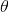: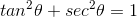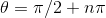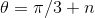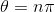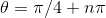Explanation:

Use the trigonometric identities to switch sec into terms of tan: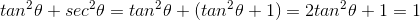hence,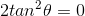So we have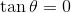, makingTherefore the solution isfor n being any integer.

Example Question #1 : Solving Trigonometric Equations And Inequalities

Which of the following is not a solution to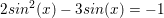for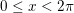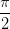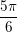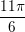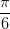Explanation:

We begin by setting the right side of the equation equal to 0.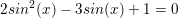The equation might be easier to factor using the following substitution.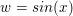This gives the following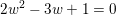This can be factored as follows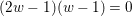Therefore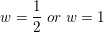Replacing our substitution therefore gives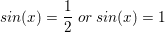Within our designated domain, we get three answers between our two equations.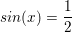when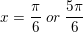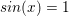when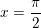Therefore, the only choice that isn't correct isExample Question #15 : Trigonometric Identities

Find one possible value of.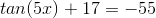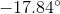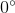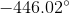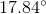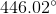Explanation:

Begin by isolating the tangent side of the equation: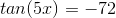Next, take the inverse tangent of both sides: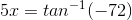Divide by five to get the final answer: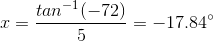Example Question #16 : Trigonometric Identities

Use trigonometric identities to solve for the angle value.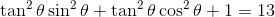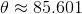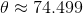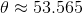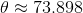Explanation:

There are two ways to solve this problem. The first involves two trigonometric identities: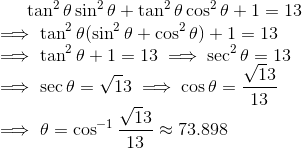The second method allows us to only use the first trigonometric identity: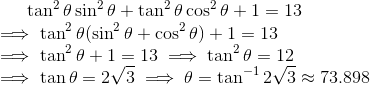Example Question #1 : Solve Trigonometric Equations And Inequalities

Use trigonometric identities to solve the equation for the angle value.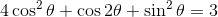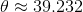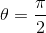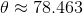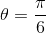Explanation:

The simplest way to solve this problem is using the double angle identity for cosine.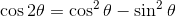Substituting this value into the original equation gives us: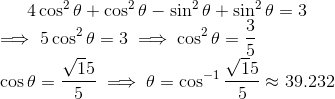All Precalculus Resources